# 1 Data Simulation

netprioR requires the following data as input

• A priori known labels for true positive (TP) and true negative (TN) genes $$Y$$
• Network data describing gene–gene similarities $$G$$
• Gene phenotypes (and other covariates) $$X$$

In the following steps we simulate data for a set of N = 1000 genes and a two class prioritisation task (positive vs. negative) and benchmark the performance of our model against the case where we prioritise soleley based on phenotypes.

## 1.1 Prior knowledge labels

We simulate the case where we know 100 labels a priori, which corresponds to 10% labelled data. We simulate equal numberos of 50 positives and 50 negatives, setting

members_per_class <- c(N/2, N/2) %>% floor

Then we simulate the labels, randomly choosing equal numbers of a priori known labels for each class.

class.labels <- simulate_labels(values = c("Positive", "Negative"), sizes = members_per_class,
nobs = c(nlabel/2, nlabel/2))

The list of simulated labels contains the complete vector of 1000 labels labels.true and the vector of observed labels labels.obs. Unknown labels are set to NA.

names(class.labels)
 "labels.true" "labels.obs"  "labelled"    "unlabelled" 

## 1.2 Network data

Next, we simulate high noise and low noise network data in the form of 1000 x 1000 adjacency matrices. The low noise networks obey the class structure defined above, whereas the high noise networks do not.

networks <- list(LOW_NOISE1 = simulate_network_scalefree(nmemb = members_per_class,
pclus = 0.8), LOW_NOISE2 = simulate_network_scalefree(nmemb = members_per_class,
pclus = 0.8), HIGH_NOISE = simulate_network_random(nmemb = members_per_class,
nnei = 1))

The networks are sparse binary adjacency matrices, which we can visualise as images. This allows to see the structure within the low noise networks, where we observe 80% of all edges in the 2nd and 4th quadrant, i.e. within each class, as defined above.

image(networks$LOW_NOISE1)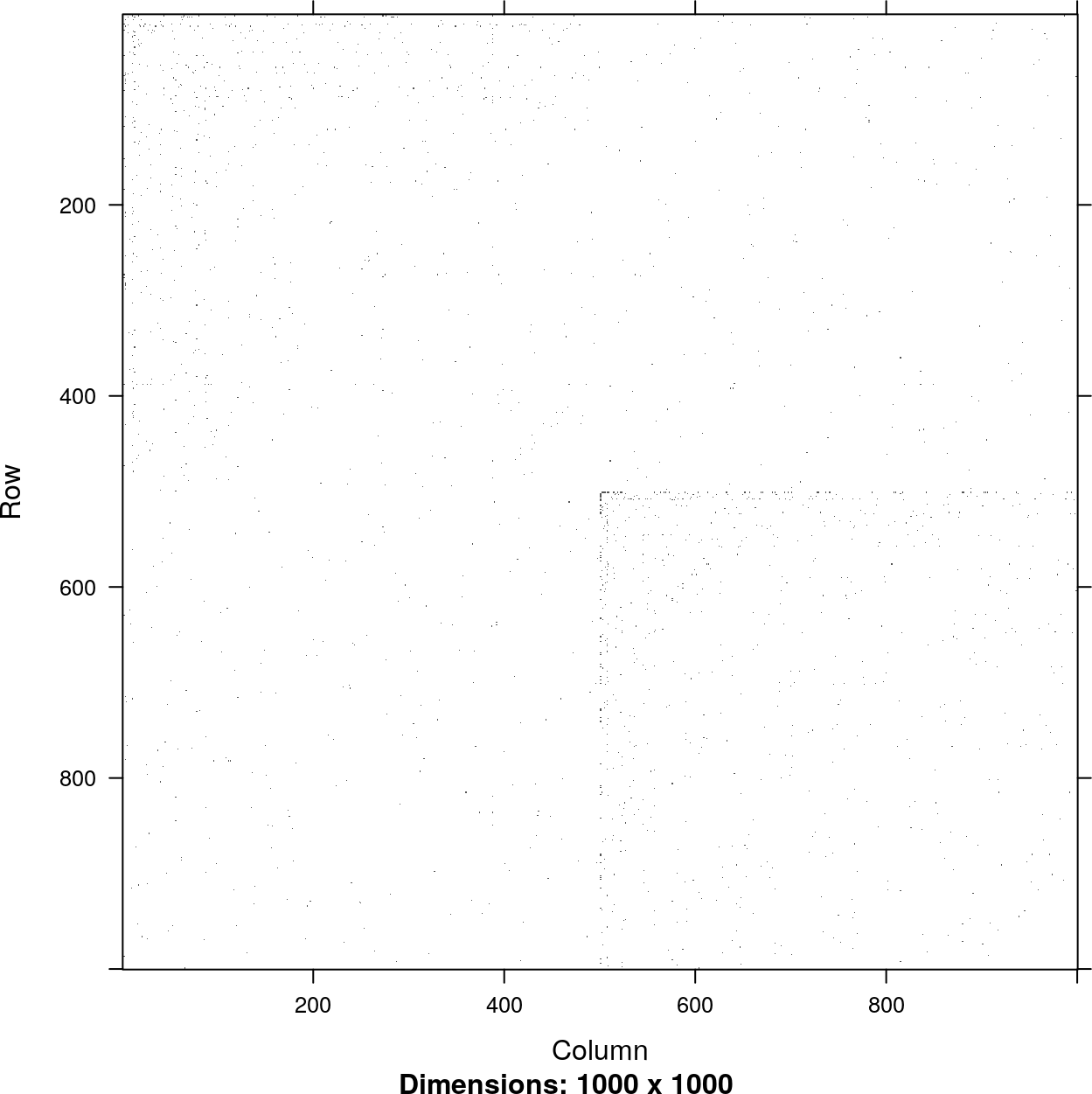## 1.3 Phenotypes We simulate phenotype matching our simulated labels from two normal distributions with a difference in means that reflects our phenotype effect size. We set the effect size to effect_size <- 0.25 and simulate the phenotypes phenotypes <- simulate_phenotype(labels.true = class.labels$labels.true, meandiff = effect_size,
sd = 1)

The higher the phenotype effect size is, the easier it is to separate the two classes soleley based on the phenotype. We visualise the phenotypes for the two classes as follows

data.frame(Phenotype = phenotypes[, 1], Class = rep(c("Positive", "Negative"),
each = N/2)) %>% ggplot() + geom_density(aes(Phenotype, fill = Class), alpha = 0.25,
adjust = 2) + theme_bw()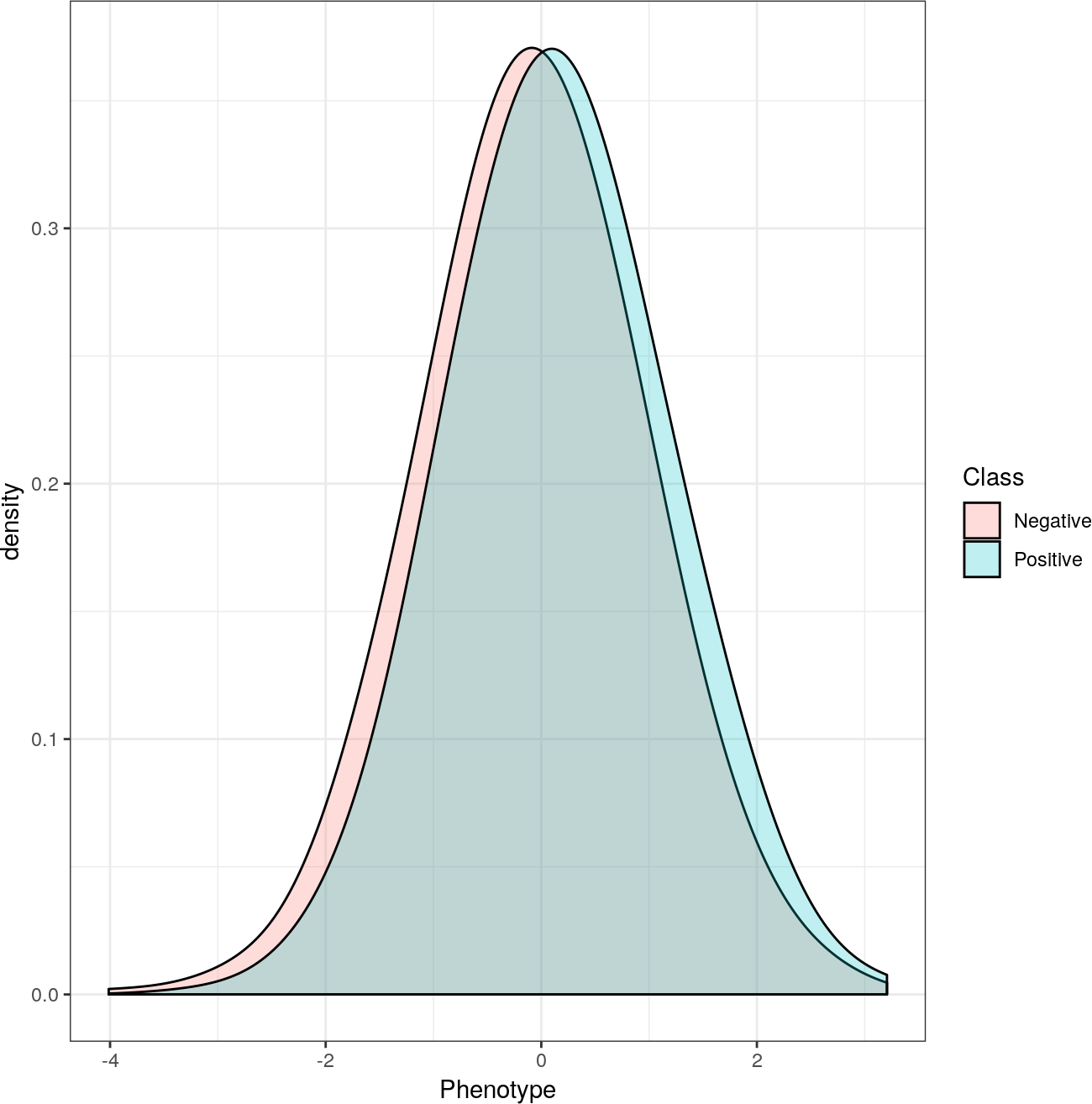# 2 Gene Prioritisation

Based on the simulated data above, we now fit the netprioR model for gene prioritisation. In this example, we will use hyperparameters a = b = 0.01 for the Gamma prior of the network weights in order to yield a sparsifying prior We will fit only one model, setting nrestarts to 1, whereas in practise multiple restarts are used in order to avoid cases where the EM gets stuck in local minima. The convergence threshold for the relative change in the log likelihood is set to 1e-6.

np <- netprioR(networks = networks, phenotypes = phenotypes, labels = class.labels$labels.obs, nrestarts = 1, thresh = 1e-06, a = 0.1, b = 0.1, fit.model = TRUE, use.cg = FALSE, verbose = FALSE) We can investigate the netprioR object using the summary() function. summary(np) #Genes: 1000 #Networks: 3 #Phenotypes: 1 #Labels: 100 Classes: Negative Positive Model: Likelihood[log]: -2951.133 Fixed effects: -0.0323836 Network weights: Network Weight LOW_NOISE1 439.28305 LOW_NOISE2 483.54036 HIGH_NOISE 49.36721 It is also possible to plot the netprioR object to get an overview of the model fit. plot(np, which = "all")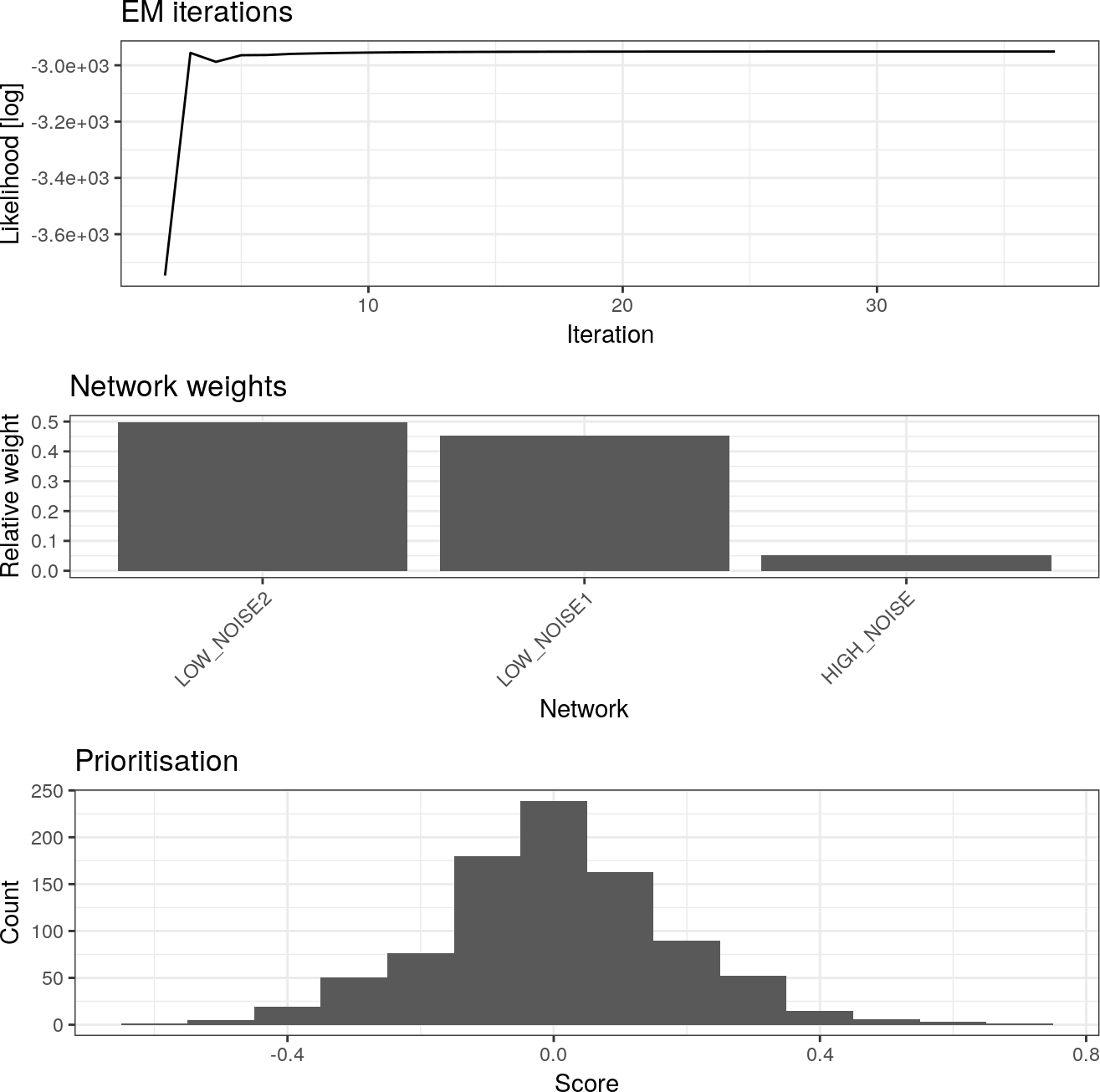We can also plot individual plots by setting which = "weights|ranks|lik". # 3 Performance evaluation We can see that the relative weight of the low noise networks is much higher than for the high noise networks indicating that, as expected, the low noise networks are more informative for the learning task. Second, we evaluate the performance of the prioritised list of genes by comparing the imputed, missing labels Yimp[u] against the true underlying labels Y and computing receiver operator characteristic (ROC) roc.np <- ROC(np, true.labels = class.labels$labels.true, plot = TRUE, main = "Prioritisation: netprioR")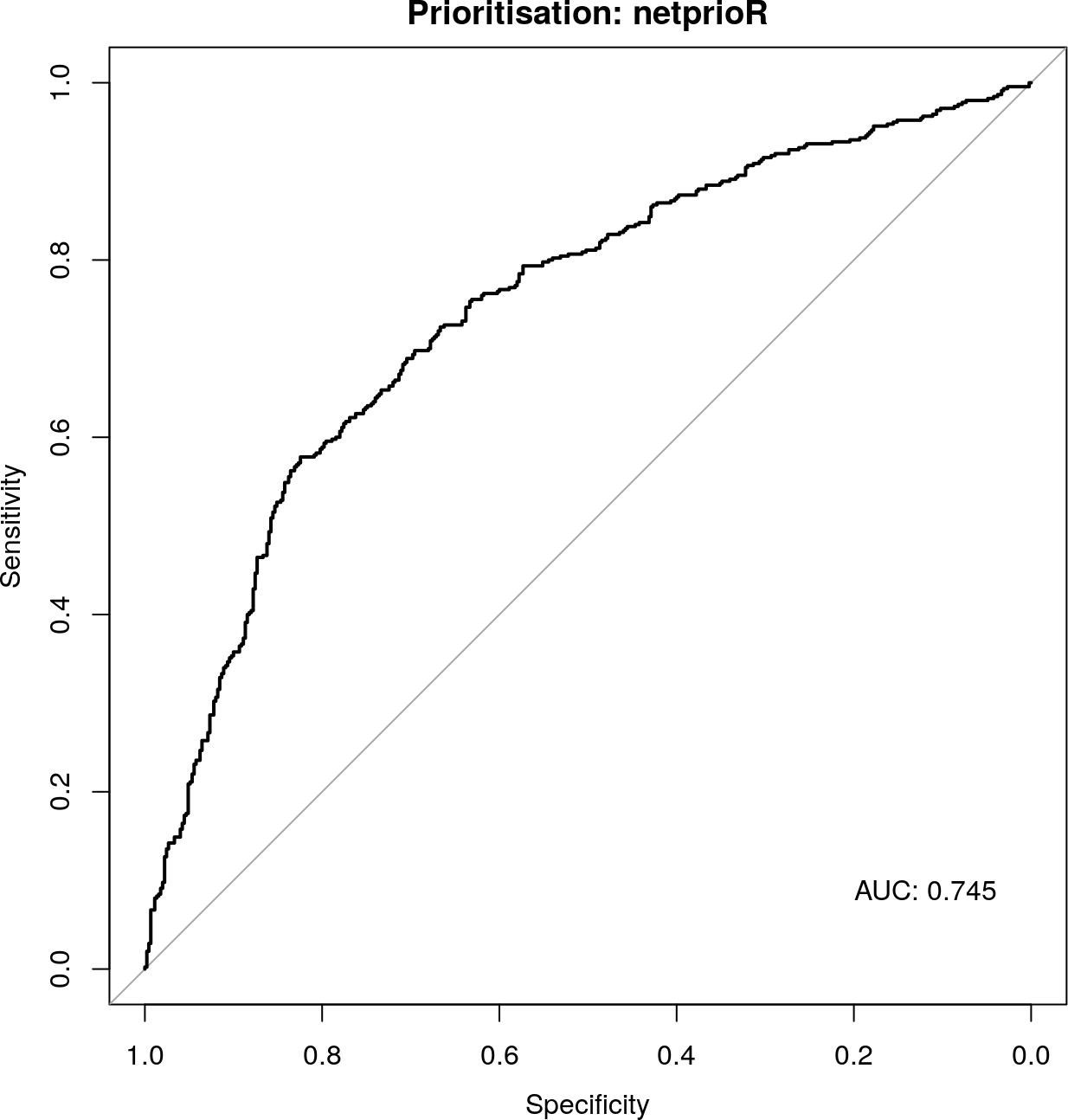In addition, we compute the ROC curve for the case where we prioritise soleley based on the phenotype

unlabelled <- which(is.na(class.labels$labels.obs)) roc.x <- roc(cases = phenotypes[intersect(unlabelled, which(class.labels$labels.true ==
levels(class.labels$labels.true))), 1], controls = phenotypes[intersect(unlabelled, which(class.labels$labels.true == levels(class.labels\$labels.true))), 1],
direction = ">")
plot.roc(roc.x, main = "Prioritisation: Phenotype-only", print.auc = TRUE, print.auc.x = 0.2,
print.auc.y = 0.1)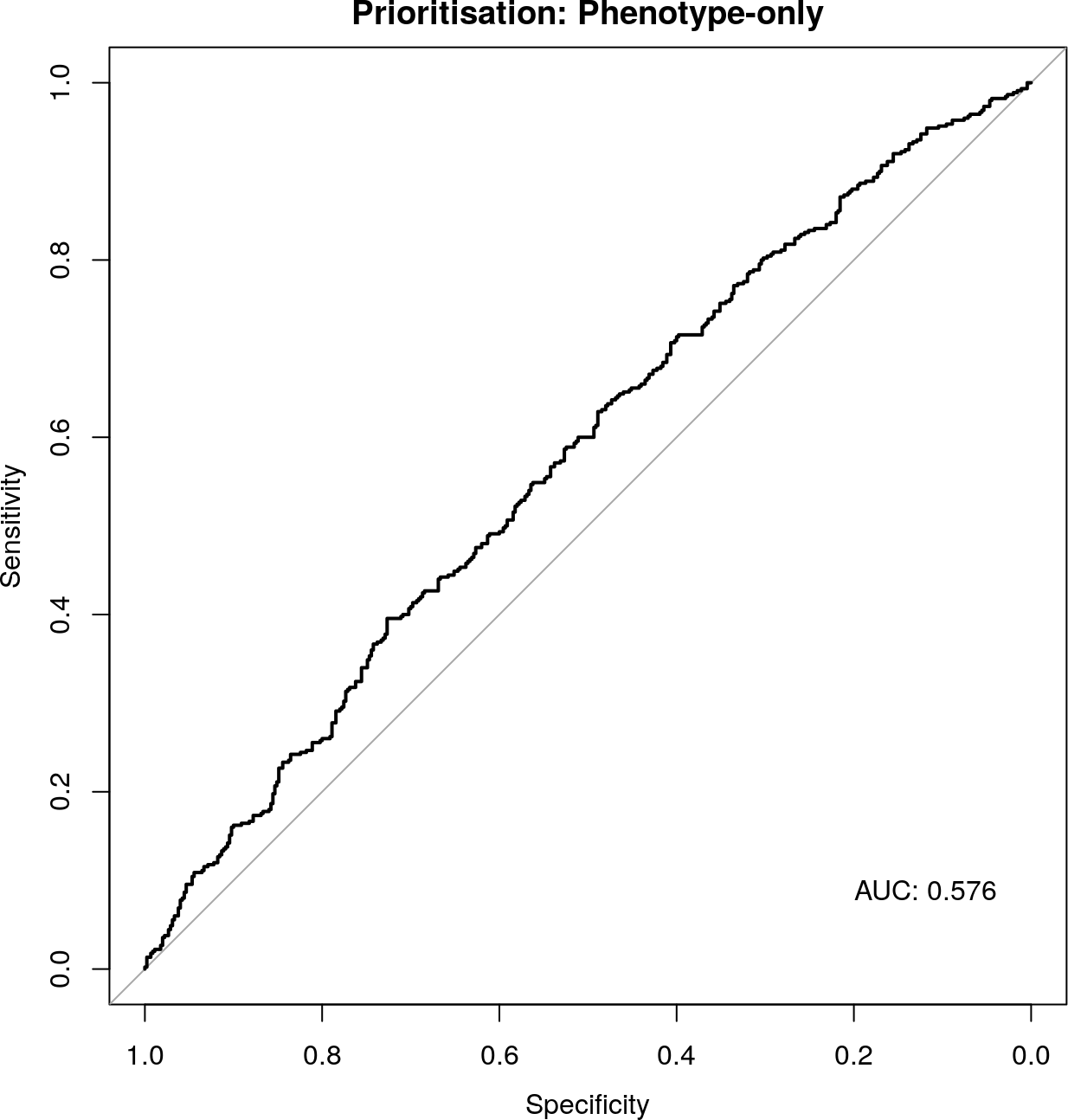Comparing the area under the receiver operator characteristic curve (AUC) values for both cases, we can see that by integrating network data and a priori known labels for TPs and TNs, we gain about 0.17 in AUC.

# 4 Session Information

Here is the output of sessionInfo() on the system on which this document was compiled:

R version 3.6.0 (2019-04-26)
Platform: x86_64-pc-linux-gnu (64-bit)
Running under: Ubuntu 18.04.2 LTS

Matrix products: default
BLAS:   /home/biocbuild/bbs-3.9-bioc/R/lib/libRblas.so
LAPACK: /home/biocbuild/bbs-3.9-bioc/R/lib/libRlapack.so

locale:
 LC_CTYPE=en_US.UTF-8       LC_NUMERIC=C
 LC_TIME=en_US.UTF-8        LC_COLLATE=C
 LC_MONETARY=en_US.UTF-8    LC_MESSAGES=en_US.UTF-8
 LC_PAPER=en_US.UTF-8       LC_NAME=C
 LC_MEASUREMENT=en_US.UTF-8 LC_IDENTIFICATION=C

attached base packages:
 stats     graphics  grDevices utils     datasets  methods   base

other attached packages:
 Matrix_1.2-17    pROC_1.14.0      netprioR_1.10.0  ggplot2_3.1.1
 pander_0.6.3     dplyr_0.8.0.1    knitr_1.22       BiocStyle_2.12.0

loaded via a namespace (and not attached):
 Rcpp_1.0.1         formatR_1.6        pillar_1.3.1
 compiler_3.6.0     BiocManager_1.30.4 plyr_1.8.4
 iterators_1.0.10   tools_3.6.0        digest_0.6.18
 evaluate_0.13      tibble_2.1.1       gtable_0.3.0
 lattice_0.20-38    pkgconfig_2.0.2    rlang_0.3.4
 foreach_1.4.4      parallel_3.6.0     yaml_2.2.0
 xfun_0.6           gridExtra_2.3      withr_2.1.2
 stringr_1.4.0      grid_3.6.0         tidyselect_0.2.5
 glue_1.3.1         R6_2.4.0           sparseMVN_0.2.1.1
 rmarkdown_1.12     bookdown_0.9       purrr_0.3.2
 magrittr_1.5       codetools_0.2-16   scales_1.0.0
 htmltools_0.3.6    assertthat_0.2.1   colorspace_1.4-1
 labeling_0.3       stringi_1.4.3      lazyeval_0.2.2
 munsell_0.5.0      doParallel_1.0.14  crayon_1.3.4